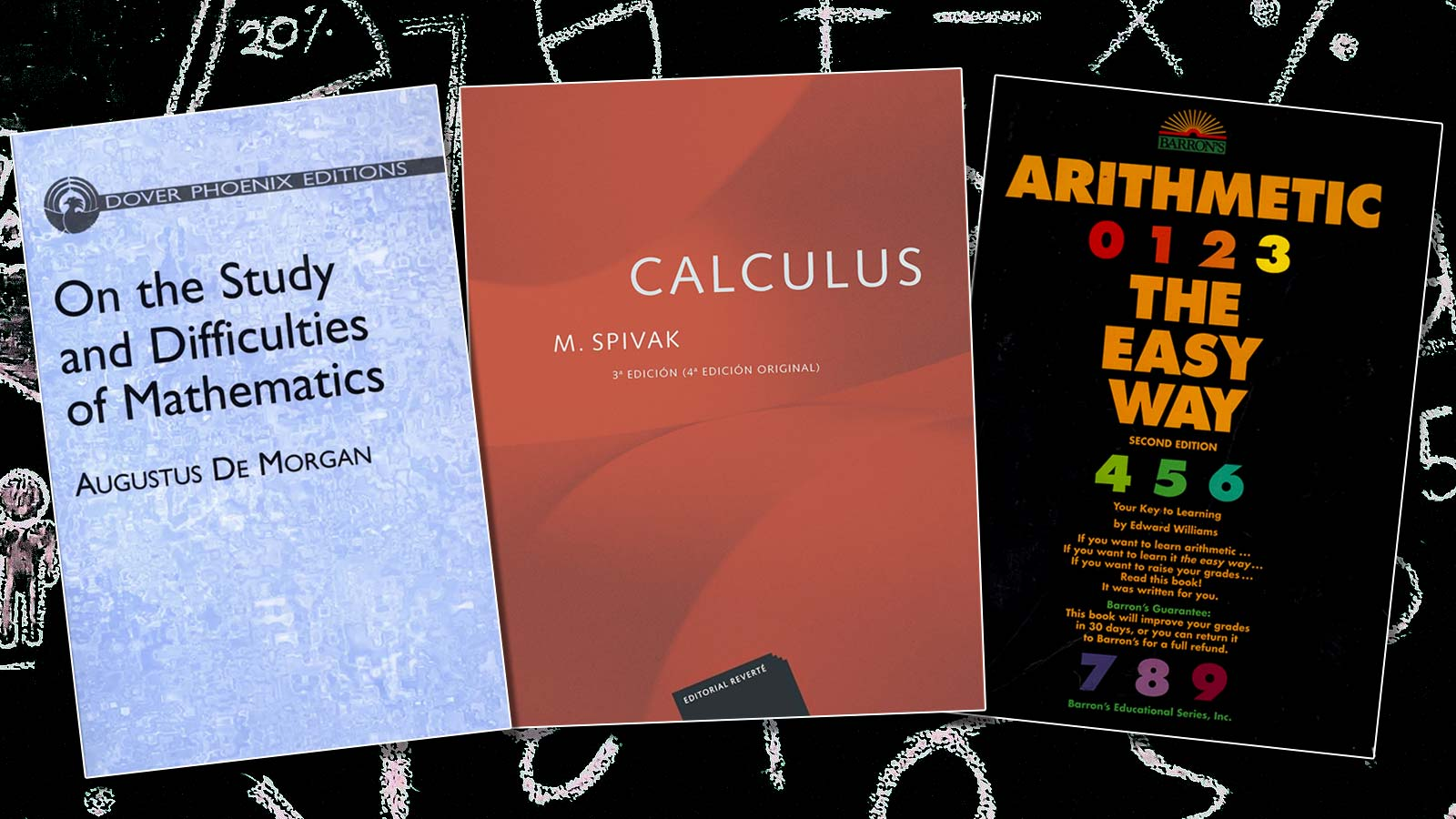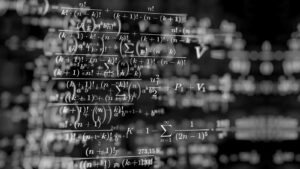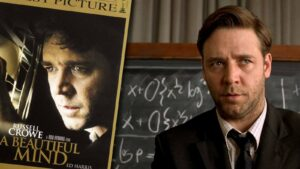You might be a student or any individual who loves reading and writing. Whether it’s reviews, essays, or stories, you have absolutely no problems writing them. They’re so easy for you wouldn’t mind helping out teens with their academic papers. However, when it comes to math, you’re extremely scared. Physics, Chemistry, Economics, or any of the other subjects that use math is a nightmare for you.

Sometimes you wish that all those formulas and concepts were easy to understand. So, we’ve decided to help you out with some books to overcome your fear of math. They’ll be extremely useful in learning and exploring various aspects of this subject. Now, let’s go through the best three books on mathematics:

## 1. On The Study and Difficulties of Mathematics

Augustus De Morgan was one of the prominent writers in mathematics during the 20th century. Among his best works is On The Study And Difficulties Of Mathematics. It consists of points that are natural and fresh in their tone. You’ll find the book quite different from the mechanical textbooks that are currently in use for studying the subject.

It presumes that the reader only knows the rules of Euclidean theorems and algebra. De Morgan gives some introductory remarks in the beginning on the nature and objects of mathematics. Then he goes on to discuss arithmetical notion and the elementary rules of the concept. This includes decimal fractions and arithmetical reactions.

He reviews the elementary principles on algebra and examines the first and second degree equations. Moving further, he also surveys logarithms and roots. In the concluding parts of the book, De Morgan explores geometrical reasoning encompassing the formulation and usage of axioms. Along with this he explores the role of proportion and several applications of algebra.

## 2. Arithmetic the Easy Way

This book by Edward Williams serves as an easy guide for general readers who wish to learn mathematics. It will help you master the basic math skills you need if you’re a student. This book gives students their much-needed foundation before they move on to high-school level math.

It can also be used as a reference for those who’ve moved beyond high school. The book begins with the basics of addition, subtraction, multiplication, and division. Then it moves further towards calculating with decimals, fractions, and percentages. In the concluding parts, the author has dedicated a chapter to reviewing the units of measurement and word problems.

You’ll find many practice exercises in the chapters and their answers given in the ending pages of the book. Arithmetic The Easy Way also features several charts, tables, and other illustrations.

## 3. Calculus

With this book, Michael Spivak aims to help students who are just getting used to proof-based mathematics. This is his celebrated textbook on mathematics. It is widely regarded as one of the finest of all introductions to mathematical analysis. You’ll learn how logical reasoning along with the fundamental concepts can make a rigorous mathematical theory.

Students have traditionally found it difficult to grasp the topic of analysis. In this book, Spivak offers excellent explanations of mathematical concepts. He also gives some extremely useful examples, a range of exercises, and many illustrations. This book explains everything in an easy-going approach.

Many hold Calculus in high regard as a modern classic in the field of mathematics. This book would also be ideal for those studying for their honors and mathematical majors.Previous post A Brief Overview of the Advancement of MathematicsNext post 6 Films That Portrayed Genius Mathematicians